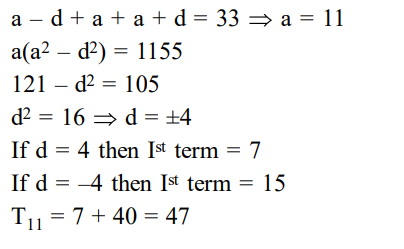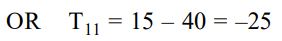# If the sum and product of the first three term in an A.P`
Question:

If the sum and product of the first three term in an A.P. are 33 and 1155 , respectively, then a value of its $11^{\text {th }}$ term is :-

1. $-25$

2. 25

3. $-36$

4. $-35$

Correct Option: 1

Solution: